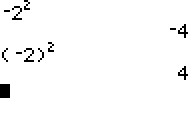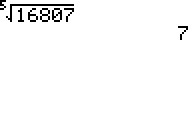# Knowledge Base

## Solution 11718: Calculating nth Powers and nth Roots Using the TI-83 Family, TI-84 Plus Family, and TI-Nspire™ Handheld in TI-84 Plus Mode.

### How do I calculate nth powers and nth roots using the TI-83 family, TI-84 Plus family, and TI-Nspire handheld in TI-84 Plus mode?

To calculate nth powers and nth roots using the TI-83 family, TI-84 Plus family, and TI-Nspire handheld in TI-84 Plus Mode, follow the examples below.

Please Note: All screenshots contained in this Knowledge Base solution were created using the TI-84 Plus family Operating System v 2.55MP

nth Powers

To raise a number by an exponent, use the caret (^) key.

Example: 3 = ?

• Input 3.
• Press [^].
• Input 4 and press [ENTER] to complete the calculation.Example: -22  = ?

• Press [ ( ] [(-)].

NOTE: The [(-)] key is called the negate key and is located below the  key.

• Input 2.
• Press [ ) ].
• Press [^].
• Input 2 and press [ENTER] to complete the calculation.

NOTE: Since the handhelds evalute the negate symbol as multiplying the value by (-1), and exponents come before multiplication using the Order of Operations, the handhelds will evaluate -2as (-4). Thus, negative values will need to be enclosed in parentheses to compute the desired solution of 4.nth Roots

To find the nth root of a number, use the nth root command (x√). To input the nth root command, press [MATH] .

Example: Find the 5th root of 16807.

• Input 5.
• Press [MATH]  to input the nth root command.
• Input 16807 and press [ENTER] to complete the calculation.Please see the TI-83 family and TI-84 Plus family guidebooks for additional information.

TI-Nspire handheld in TI-84 Plus mode users may refer to the TI-84 Plus family guidebook.